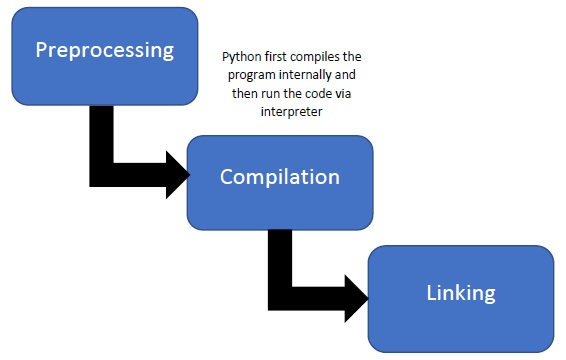# What is the process of compilation and linking in python?

Compilation − The source code in python is saved as a .py file which is then compiled into a format known as byte code, byte code is then converted to machine code. After the compilation, the code is stored in .pyc files and is regenerated when the source is updated. This process is known as compilation.

Linking − Linking is the final phase where all the functions are linked with their definitions as the linker knows where all these functions are implemented. This process is known as linking.image compilation.jpg-----

Note − Python programs are both compiled as well as interpreted, but the compilation part is hidden from the programmer. Therefore, we generally say, Python is an interpreted language for the same reason.

Let’s see an example. For our example, we will use the dis module in Python.

## Install and Import the dis module

To install the dis module, use the pip −

pip install dis


To import the dis module −

import dis


## Example

Let us now see an example

import dis

# Function to return the sum of recursive numbers
def recursive_sum(n):
if n <= 1:
return n
else:
return n + recursive_sum(n-1)

# change this value for a different result
number = 16

if number < 0:
print("The sum = ",recursive_sum(number))

# By using dis module, the bytecode is loaded into machine code, and a piece of code that reads each instruction in the bytecode and executes whatever operation is indicated.
dis.dis(recursive_sum)


### Output

  5           0 LOAD_FAST                0 (n)
4 COMPARE_OP               1 (<=)
6 POP_JUMP_IF_FALSE       12

10 RETURN_VALUE

8     >>   12 LOAD_FAST                0 (n)